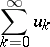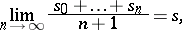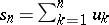# Arithmetical averages, summation method of

(diff) ← Older revision | Latest revision (diff) | Newer revision → (diff)

One of the methods for summing series and sequences. The seriesis summable by the method of arithmetical averages to the sumifwhere. In this case, one also says that the sequenceis summable by the method of arithmetical averages to the limit. The summation method of arithmetical averages is also called the Cesàro summation method of the first order (cf. Cesàro summation methods). The summation method of arithmetical averages is completely regular (see Regular summation methods) and translative (see Translativity of a summation method).

How to Cite This Entry:
Arithmetical averages, summation method of. Encyclopedia of Mathematics. URL: http://encyclopediaofmath.org/index.php?title=Arithmetical_averages,_summation_method_of&oldid=18557
This article was adapted from an original article by I.I. Volkov (originator), which appeared in Encyclopedia of Mathematics - ISBN 1402006098. See original article